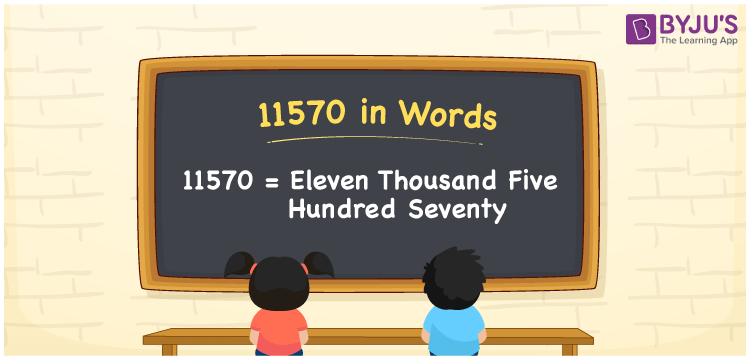# 11570 in Words

11570 in words is written as Eleven thousand five hundred seventy. In both the International System of Numerals and the Indian System of Numerals, 11570 is written as Eleven thousand five hundred seventy. The number 11570 is a Cardinal Number as it represents some quantity. For example, “that mobile phone costs 11570 rupees”.

 11570 in Words Eleven thousand five hundred seventy Eleven thousand five hundred seventy in numerical form 11570

## 11570 in English Words

11570 in English words is read as “Eleven thousand five hundred seventy”## How to Write 11570 in Words?

To write 11570 in words, we shall use the place value chart. In the place value chart, put 1 in the ten thousands and thousands, 5 in the hundreds, 7 in the tens and 0 in the ones, respectively. Let us make a place value chart to write the number 11570 in words.

 Ten Thousands Thousands Hundreds Tens Ones 1 1 5 7 0

Thus, we can write the expanded form as

1 × Ten Thousand + 1 × Thousand + 5 × Hundred + 7 × Ten + 0 × One

= 1 × 10000 + 1 × 1000 + 5 × 100 + 7 × 10 + 0 × 1

= 10000 + 1000 + 700 +70 + 0

= 11570

= Eleven thousand five hundred seventy.

11570 is a natural number, the successor of 11569 and the predecessor of 11571.

11570 in words – Eleven thousand five hundred seventy

• Is 11570 an odd number? – No
• Is 11570 an even number? – Yes
• Is 11570 a perfect square number? – No
• Is 11570 a perfect cube number? – No
• Is 11570 a prime number? – No
• Is 11570 a composite number? – Yes

## Frequently Asked Questions on 11570 in Words

Q1

### How to write 11570 in words?

11570 in words is written as Eleven thousand five hundred seventy.
Q2

### How to write 11570 in the International and Indian System of Numerals?

In both, the system of numerals, 11570 in words, is written as Eleven thousand five hundred seventy.
Q3

### How to write 11570 in a place value chart?

In the place value chart, write 1 in the ten thousands and thousands, 5 in the hundreds, 7 in the tens and 0 in the ones, respectively.
Test your Knowledge on 11570 in Words